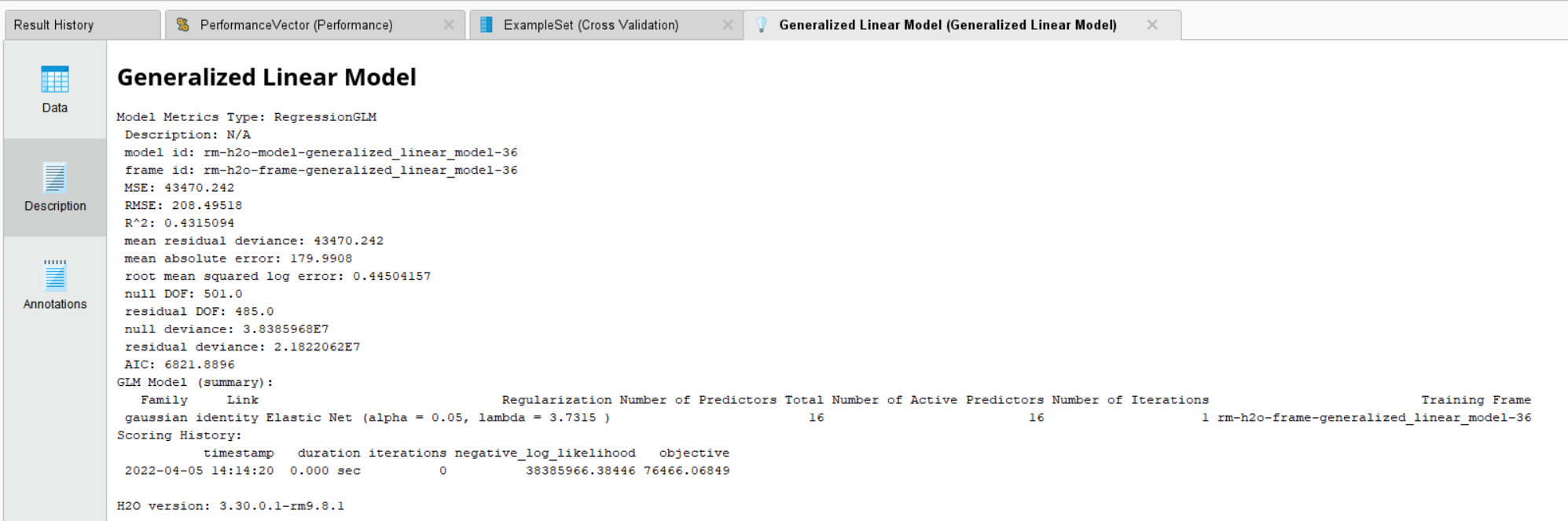# Extract regression equation

Member Posts: 3Newbie
edited April 2022 in Help
Hi,
I'm building a model to predict a numeric continuous variable by using another numeric variable.
Logarithmic or polynomial equations better fit my data. They have given a lower error rate than linear regression, but I'm unsure how to extract the model equation (formula) from the model I built.
Here is the algorithm I used:
- Generalized Linear Model
- family: gaussian
(Green label = prediction)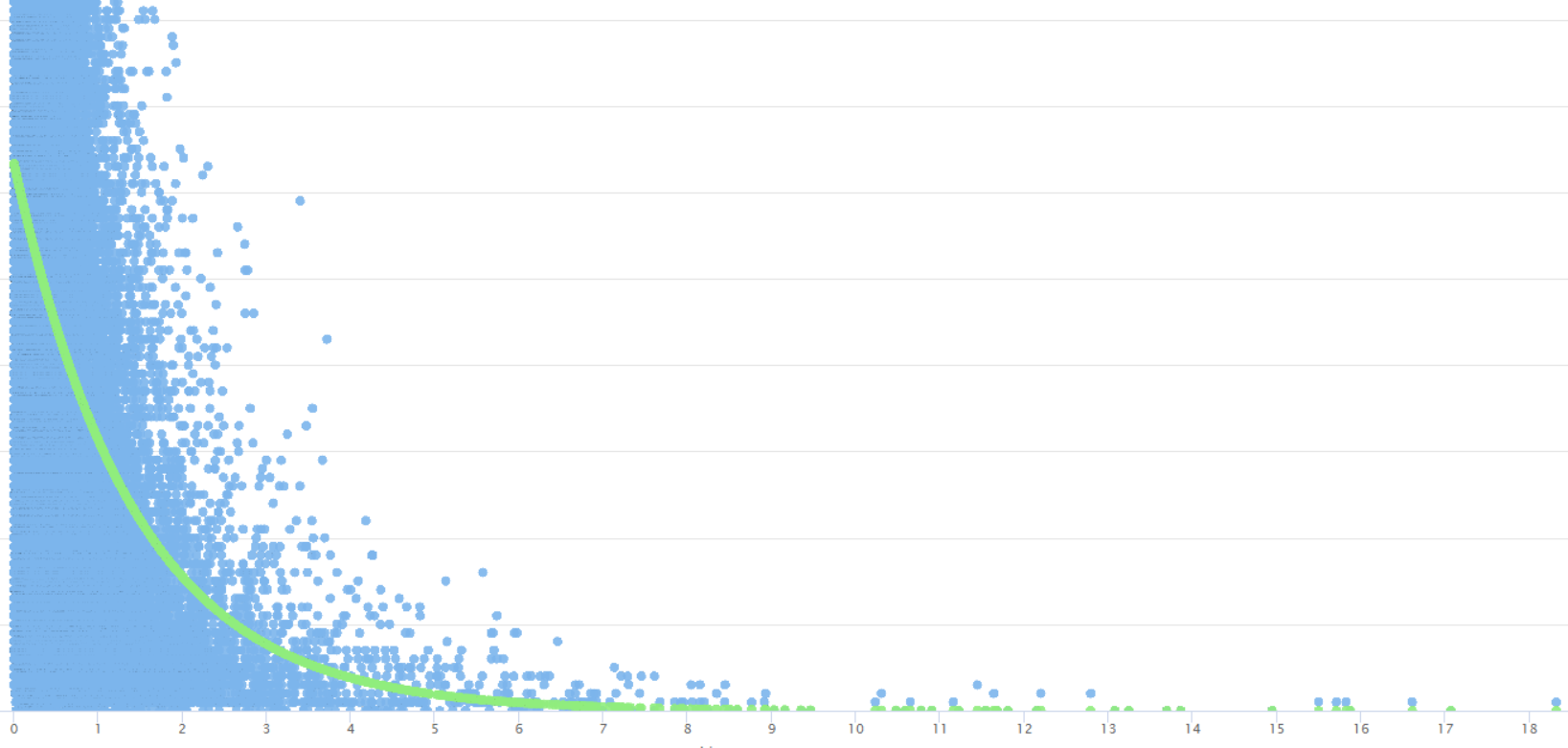Also, I am trying to use polynomial regression for my model since it shows the equation in the model output. However, the prediction is totally off, not sure if I made any mistake in the setting. (Green label = prediction)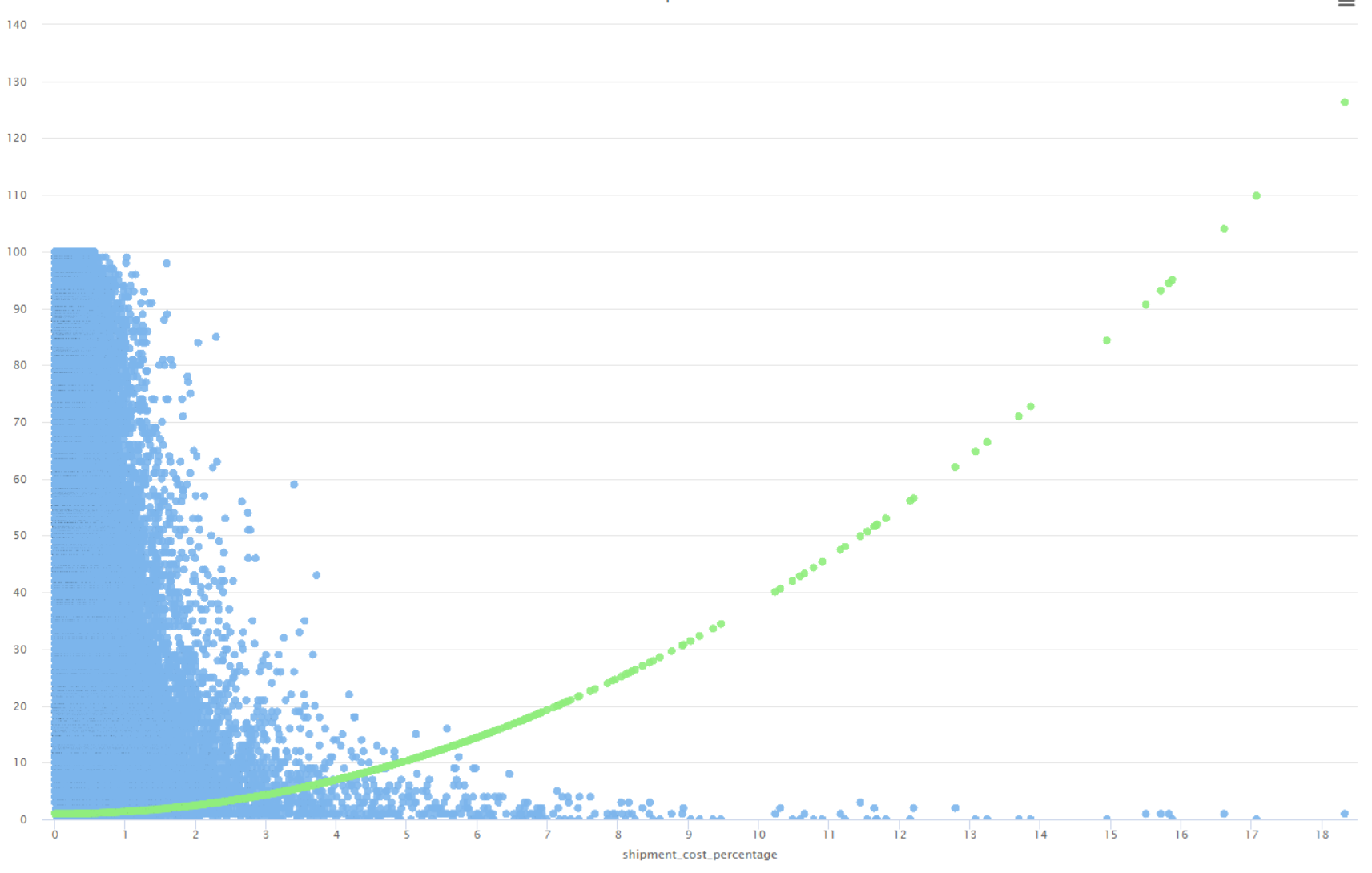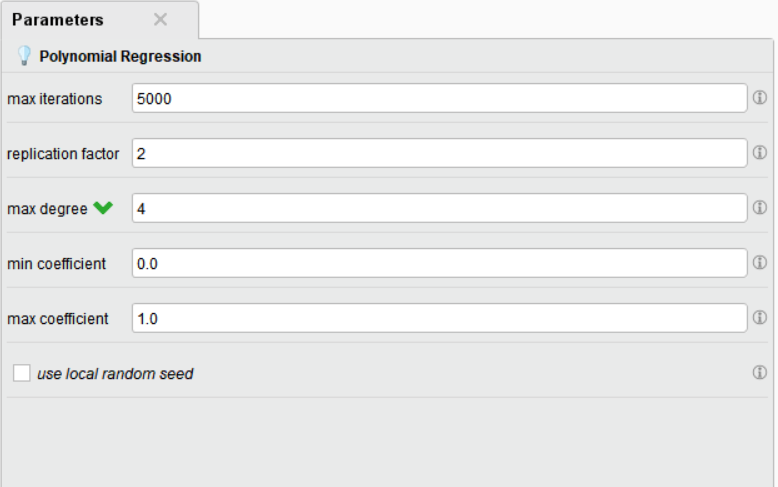Thanks,

• Member Posts: 473Unicorn
Solution Accepted
Hi @Louie
If you click on the data option into the left menu, you can see a table with the attributes and coefficients. You can construct the model with the more significant variables using the p-value  and significance level as  criteria.

The attached image is from sample process for GLM regression.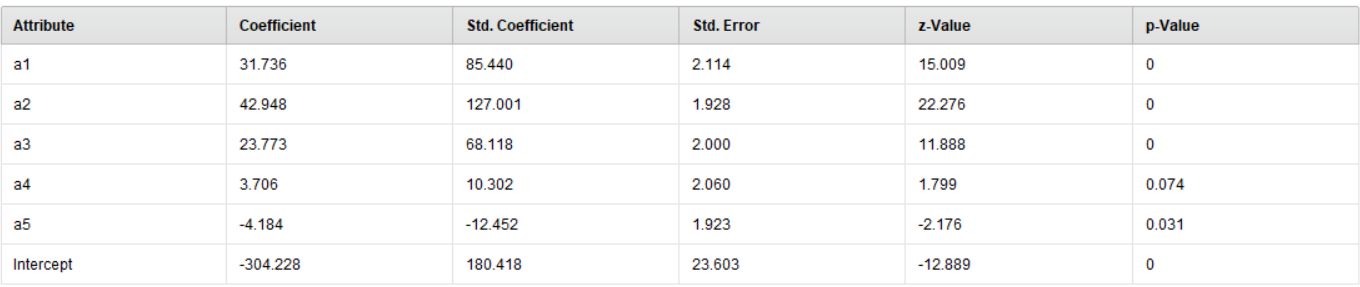Best,

Cesar

• Member Posts: 473Unicorn
Hi @Louie

There is a good guide about the use and interpretation of GLM. It was very useful for me.

https://statisticsbyjim.com/regression/interpret-coefficients-p-values-regression/

Best,

Cesar
• Member Posts: 3Newbie
edited April 2022
Hi ceaperez,
Thank you for the reference.
I understand the GLM formula and interpretation. However, I'm not sure where to find the model equation I built which I am planning to apply the model into other application (by using the formula) to predict new testing data (e.g., label = a + b(x) + c (x)   or label = a+b log (x)
. Also, I am wondering if the rapidminer GLM could automatically generate interaction terms when I have 2 or more numeric or binomial independent variables?
Thanks,
• Administrator, Moderator, Employee, RapidMiner Certified Analyst, RapidMiner Certified Expert, University Professor Posts: 3,453RM Data Scientist
Hi,
maybe you want to check the Function Fitting operator.
cheers,
Martin
- Sr. Director Data Solutions, Altair RapidMiner -
Dortmund, Germany
• Member Posts: 3Newbie
Thanks mschmitz,

The Function Fitting operator could help me for generating interaction terms.
However. I'm still not sure how to directly out up the equation from an GLM .

For example, I can find the equation directly from linear or polynomials model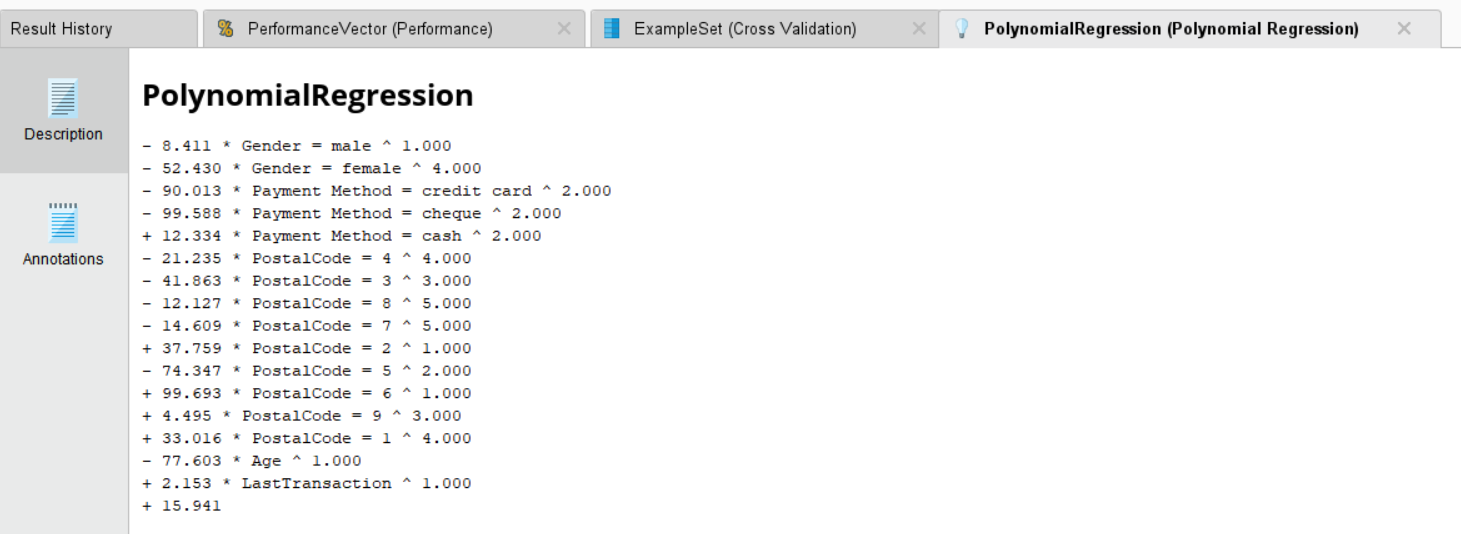but not with GLM